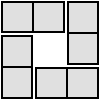#### You may also like### Roll These Dice

Roll two red dice and a green dice. Add the two numbers on the red dice and take away the number on the green. What are all the different possible answers?### Domino Square

Use the 'double-3 down' dominoes to make a square so that each side has eight dots.### 4 Dom

Use these four dominoes to make a square that has the same number of dots on each side.

# Consecutive Numbers

##### Age 7 to 14Challenge Level

Having chosen your four consecutive numbers you place $+$ and $-$ signs in between them.

You have three empty spaces, so how many possible combinations are there for arranging the $+$ and $-$ signs?

Are you sure you have found them all? How will you know?